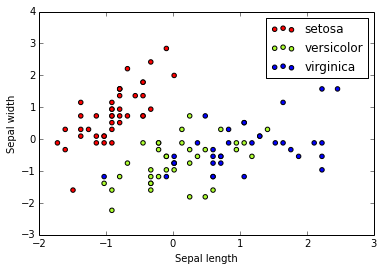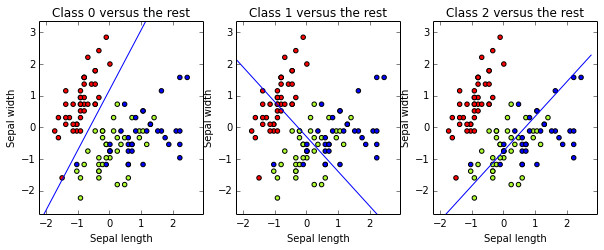# Learning Scikit-learn: Machine Learning in Python¶

## IPython Notebook for Chapter 1: A Gentle Introduction to Machine Learning¶

In this chapter, we show (using a very simple example) the general form of training and evaluating a classifier, using scikit-learn. We will use the Iris flower dataset, introduced in 1936 by Sir Ronald Fisher to show how a statistical method (discriminant analysis) worked.

Start by importing numpy, scikit-learn, and pyplot, the Python libraries we will be using in this chapter. Show the versions we will be using (in case you have problems running the notebooks).

In :
%pylab inline
import IPython
import sklearn as sk
import numpy as np
import matplotlib
import matplotlib.pyplot as plt

print 'IPython version:', IPython.__version__
print 'numpy version:', np.__version__
print 'scikit-learn version:', sk.__version__
print 'matplotlib version:', matplotlib.__version__

Populating the interactive namespace from numpy and matplotlib
IPython version: 2.1.0
numpy version: 1.8.2
scikit-learn version: 0.15.1
matplotlib version: 1.3.1


Every method implemented on scikit-learn assumes that data comes in a dataset. Scikit-learn includes a few well-known datasets. The Iris flower dataset includes information about 150 instances from three different Iris flower species, including sepal and petal length and width. The natural task to solve using this dataset is to learn to guess the Iris species knowing the sepal and petal measures. Let's import the dataset and show the values for the first instance:

In :
from sklearn import datasets
X_iris, y_iris = iris.data, iris.target
print X_iris.shape, y_iris.shape
print X_iris, y_iris

(150, 4) (150,)
[ 5.1  3.5  1.4  0.2] 0


The dataset includes 150 instances, with 4 attributes each. Our first step will be to separate the dataset into to separate sets, using 75% of the instances for training our classifier, and the remaining 25% for evaluating it (and, in this case, taking only two features, sepal width and length). We will also perform feature scaling: for each feature, calculate the average, subtract the mean value from the feature value, and divide the result by their standard deviation. After scaling, each feature will have a zero average, with a standard deviation of one. This standardization of values (which does not change their distribution, as you could verify by plotting the X values before and after scaling) is a common requirement of machine learning methods, to avoid that features with large values may weight too much on the final results.

In :
from sklearn.cross_validation import train_test_split
from sklearn.preprocessing import StandardScaler

# Get dataset with only the first two attributes
X, y = X_iris[:,:2], y_iris
# Split the dataset into a trainig and a testing set
# Test set will be the 25% taken randomly
X_train, X_test, y_train, y_test = train_test_split(X, y, test_size=0.25, random_state=33)
print X_train.shape, y_train.shape
# Standarize the features
scaler = StandardScaler().fit(X_train)
X_train = scaler.transform(X_train)

X_test = scaler.transform(X_test)

(112, 2) (112,)


Let's plot the training data using pyplot

In :
colors = ['red', 'greenyellow', 'blue']
for i in xrange(len(colors)):
px = X_train[:, 0][y_train == i]
py = X_train[:, 1][y_train == i]
plt.scatter(px, py, c=colors[i])

plt.legend(iris.target_names)
plt.xlabel('Sepal length')
plt.ylabel('Sepal width')

Out:
<matplotlib.text.Text at 0x107661d10>Note that setosa is easily separable from the other two classes, while versicolor and virginica are pretty messed... To implement linear classification, we will use the SGDClassifier from scikit-learn. SGD stands for Stochastic Gradient Descent, a very popular numerical procedure to find the local minimum of a function (in this case, the loss function, which measures how far every instance is from our boundary). The algorithm will learn the coefficients of the hyperplane by minimizing the loss function. Let's fit a Linear Classification method to our training data, and show the built hyperplane:

In :
# create the linear model classifier
from sklearn.linear_model import SGDClassifier
clf = SGDClassifier()
# fit (train) the classifier
clf.fit(X_train, y_train)
# print learned coeficients
print clf.coef_
print clf.intercept_

[[-28.56232699  15.06870628]
[ -8.94402784  -8.14000854]
[ 14.04159132 -12.8156682 ]]
[-17.62477802  -2.35658325  -9.7570213 ]


Plot the three calculated decision curves. Note that Class 0 is linearly separable, while Class 1 and Class 2 are not

In :
x_min, x_max = X_train[:, 0].min() - .5, X_train[:, 0].max() + .5
y_min, y_max = X_train[:, 1].min() - .5, X_train[:, 1].max() + .5
xs = np.arange(x_min,x_max,0.5)
fig, axes = plt.subplots(1,3)
fig.set_size_inches(10,6)
for i in [0,1,2]:
axes[i].set_aspect('equal')
axes[i].set_title('Class ' + str(i) + ' versus the rest')
axes[i].set_xlabel('Sepal length')
axes[i].set_ylabel('Sepal width')
axes[i].set_xlim(x_min, x_max)
axes[i].set_ylim(y_min, y_max)
plt.sca(axes[i])
for j in xrange(len(colors)):
px = X_train[:, 0][y_train == j]
py = X_train[:, 1][y_train == j]
plt.scatter(px, py, c=colors[j])
ys = (-clf.intercept_[i]-xs*clf.coef_[i,0])/clf.coef_[i,1]
plt.plot(xs,ys,hold=True)Let's how our classifier can predict the class of a certain instance, given its sepal length and width:

In :
print clf.predict(scaler.transform([[4.7, 3.1]]))
print clf.decision_function(scaler.transform([[4.7, 3.1]]))


[[ 19.77232705   8.13983962 -28.65250296]]


Let's see how good our classifier is on our training set, measuring accuracy:

In :
from sklearn import metrics
y_train_pred = clf.predict(X_train)
print metrics.accuracy_score(y_train, y_train_pred)

0.821428571429


To get a better idea of the expected performance of our classifier on unseen data, que must measure accuracy on the testing set

In :
y_pred = clf.predict(X_test)
print metrics.accuracy_score(y_test, y_pred)

0.684210526316


Let's try some additinoal measures: Precision, Recall and F-score, and show the confusion matrix

In :
print metrics.classification_report(y_test, y_pred, target_names=iris.target_names)
print metrics.confusion_matrix(y_test, y_pred)

             precision    recall  f1-score   support

setosa       1.00      1.00      1.00         8
versicolor       0.43      0.27      0.33        11
virginica       0.65      0.79      0.71        19

avg / total       0.66      0.68      0.66        38

[[ 8  0  0]
[ 0  3  8]
[ 0  4 15]]


Now, let's try cross-validation: divide the dataset into n parts, train on n-1 and evaluate on the remaining one; do this n times and take the mean. We will, for this, create a new classifier: a pipeline of the standarizer and the linear model. Measure the cross-validation accuracy for each fold:

In :
from sklearn.cross_validation import cross_val_score, KFold
from sklearn.pipeline import Pipeline

# create a composite estimator made by a pipeline of the standarization and the linear model
clf = Pipeline([
('scaler', StandardScaler()),
('linear_model', SGDClassifier())
])
# create a k-fold croos validation iterator of k=5 folds
cv = KFold(X.shape, 5, shuffle=True, random_state=33)
# by default the score used is the one returned by score method of the estimator (accuracy)
scores = cross_val_score(clf, X, y, cv=cv)
print scores

[ 0.73333333  0.63333333  0.73333333  0.66666667  0.6       ]


Calculate the mean and standard error of cross-validation accuracy

In :
from scipy.stats import sem

def mean_score(scores):
"""Print the empirical mean score and standard error of the mean."""
return ("Mean score: {0:.3f} (+/-{1:.3f})").format(
np.mean(scores), sem(scores))

print mean_score(scores)

Mean score: 0.673 (+/-0.027)